# How to use the MATCH function in Google SheetsIn Google Sheets, the MATCH function gives you the relative position of an item within a range of cells. Basically, if you want to know the position of a specific value within a range or array, MATCH will tell you where it’s located. MATCH is handy but fairly basic, but when you combine it with INDEX, it becomes pretty powerful. The INDEX MATCH function combination is a brilliant, more dynamic alternative to the VLOOKUP function. It solves many of the problems associated with VLOOKUP.

#### MATCH

Firstly, let’s take a look at the MATCH function and how it works in Google Sheets.

Remember that MATCH returns a position but not a value, so if you want to return a value itself, use INDEX, HLOOKUP, or VLOOKUP.

In the snapshot below you can see that the position of Evan is 5 within the range of cells A1 to A6.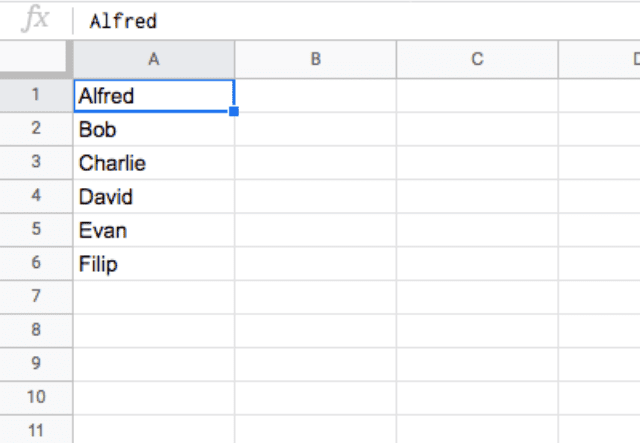What if you place the cells as shown in the snapshot below (B4 to B9)?

The relative position of Evan still remains 5.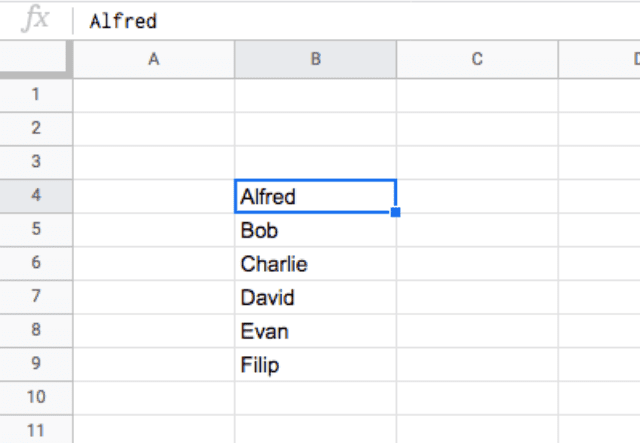That is exactly what the MATCH function is designed to do – return the relative position of an item (‘Evan’) in a range of cells (A1:A6 or B4:B9).

### Syntax

=MATCH(search_key, range, search_type)

• search_key – is the item that the MATCH function searches for within the range of cells. It can be a pure text (‘Evan’), or a cell reference (like A7), or even a function that returns a string or a number (like LEFT(“Mike Johnson”,8) or DATE(2017,1,1))
• range – is the group of cells where the MATCH function searches for the item (search_key). This must be a one-dimensional array, i.e. either a range with a single column or a single row.
• search_type – is an optional input that directs how the MATCH function should search for the search_key in the range. This takes in three different values:
• 1 is the default value (i.e. when no input is provided against search_type). Going with this option, Google Sheets assumes that the range of cells are sorted in ascending order, and accordingly returns the largest value less than or equal to search_key.
• 0 specifies Google Sheets that it must find an exact match. This is the ideal option to go with if the range of cells is not sorted in any order.
• 1, as one would guess, is the exact opposite of 1. This option assumes that the range of cells are sorted in descending order, and returns the smallest value greater than or equal to search_key.

#### How to use MATCH in Google Sheets

Take a look at the screenshot below. In column A, I have a set of data in ascending order (e.g. smallest to largest). I’ve tried out a few variations of the MATCH function on this data.

In the first example, in row 2, I’ve asked the MATCH function to search for ID #1400 by typing =Match(1400,A2:A15,1).

The second formula, in row 3, does the same as the first, and both tell me that ID #1400 is in the 7th row.

The difference is that in the first example, I used search_type 1, which is used when the data is in ascending order (which it is).

The second time, I used search_type 0, which is used when the data is not sorted, or when you only want an exact match. Because the data was sorted, and there was an exact match for 1400, both functions gave me the same result.

In the third example, in row 4, I ask the MATCH function to find me ID #1300 by typing =Match(1300,A2:A15,0). I use search_type 0 to specify that I want an exact match. Because there is no ID #1300, I get an error.

In the fourth example in row 5, however, I ask the same thing but use search_type 1. Here, the function does give me a result, indicating that the answer is in row 4.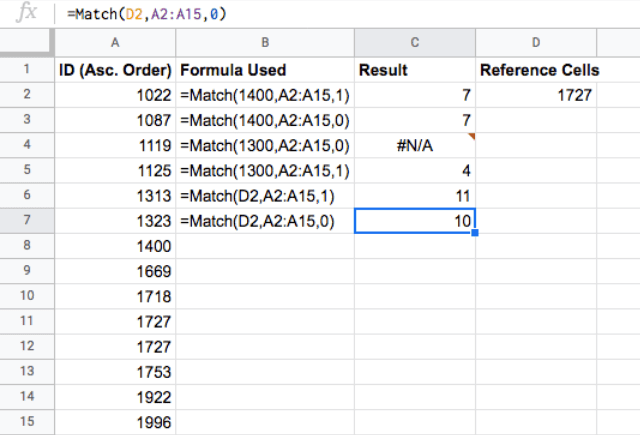One thing to remember is that the MATCH function won’t necessarily give you the closest answer — as you can see, row 4 corresponds to ID #1125.

The next row, ID #1313, is much closer to the ID #1300 I was asking for. However, when searching data in ascending order, the function returns the closest value that is no larger than the search_key. Because of this, it returns row 4 as the result, not row 5.

In the last pair of examples, in row 6 and 7, instead of typing in the search_key directly, I indicate a reference cell which contains the search_key. I do this with the function =Match(D2,A2:A15,1).

In the next example, I’ll show the data sorted in descending order.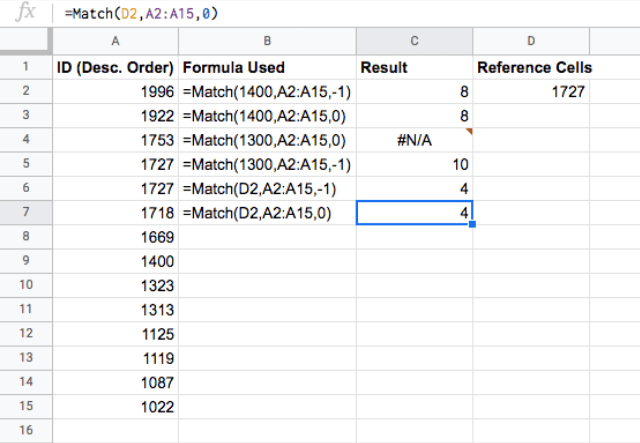The examples here are the same as in the first image, except I am now using a search_key of -1 instead of 1 to reflect the fact that the data is sorted in descending order.

Notice the example of searching for ID #1300 – this time, the function gives a result of row 10, which corresponds to ID #1313, much closer to 1300 than when the same thing was done with the data sorted in ascending order.

#### Using MATCH with text

The examples have shown have been with numeric values. How about using the MATCH function with text values?

Since you can’t exactly define the ‘less than’ and ‘greater than’ values for text forms, I usually go with the search_type 0, which tells Google Sheets to search for an exact match. Here are a few examples: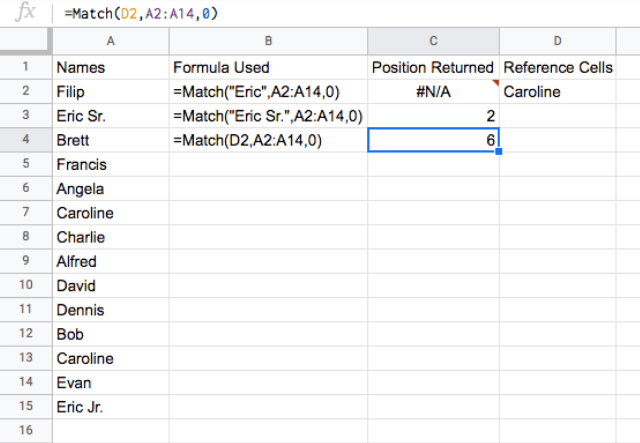Notice how the search_key needs to be in quotation marks when it is a text string, like in the first example: =Match(“Eric”,A2:A14,0). Another thing to remember is that when using search_key 0 to search for an exact match, if there is no exact match the function will give an error (like in the first example above).

I’ve been using the MATCH function in a vertical layout because that’s most common. But there are certainly times when you might want to use it for a horizontal data set. In the example below, I’ve done just that.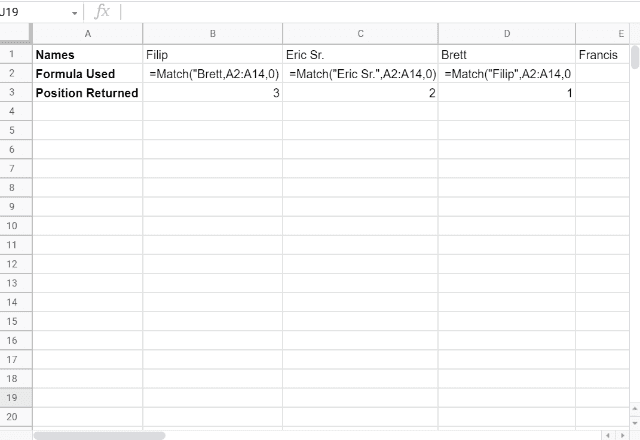Here, the MATCH function tells you the number of the column that contains the search_key.

### How to use the INDEX MATCH function combination

Perhaps the most powerful use of MATCH in Google Sheets is when you use it in combination with the INDEX function, in order to look up values. But there’s already a VLOOKUP function in Google Sheets for that purpose, right?

True — but the combination of MATCH and INDEX can solve several problems that arise when using VLOOKUP.

Take a look at the VLOOKUP example below: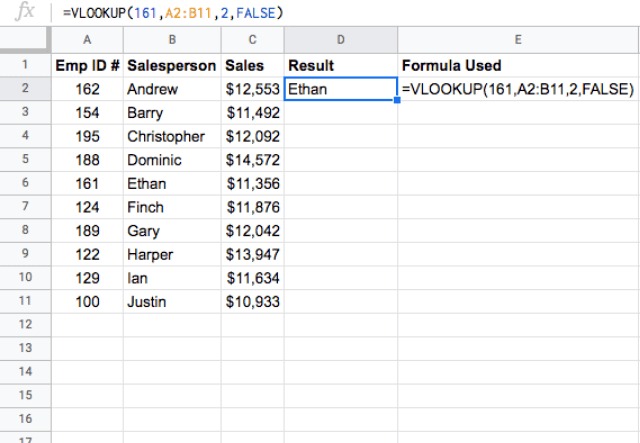The VLOOKUP function in D2 looks up 161 in the ‘Emp ID #’ column (as it’s the leftmost in the range A2:B11), and from the row where it finds the value 161, it fetches the value located in the second column (i.e. column B), while assuming the data is not sorted. So far, so good. But there are two critical problems when using VLOOKUP in Google Sheets.

#### Problem 1: Static cell referencing

What happens when you insert a new column between the first and second columns? Let’s try that.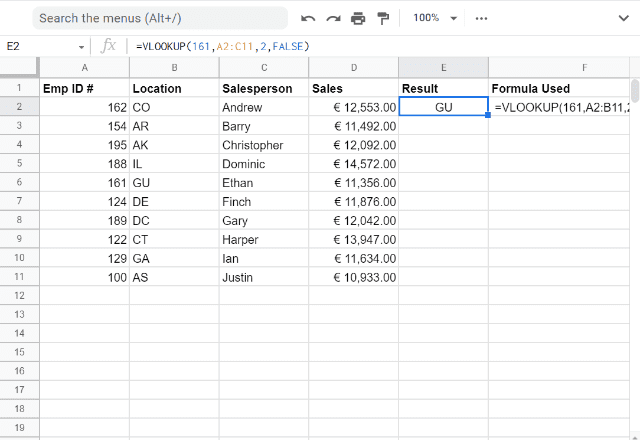You’ll notice the returned value is not Ethan anymore. This is because VLOOKUP is a semi-static function.

Google Sheets updated the second parameter to reflect the new range, but it did not accordingly change the column index (third parameter) when a new column was added before the Salesperson column.

#### Problem 2: Lookup column is always the leftmost

You might find yourself in a situation where you have to look up values from a column (Emp ID #) that is not the leftmost, as shown below.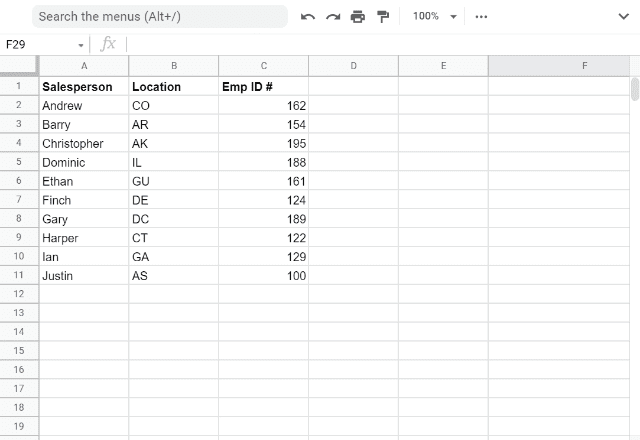In this case, moving the Emp ID # column to make it the leftmost would work. But that isn’t an ideal approach – there can be data layout or presentation specifications that do not allow you to re-arrange columns. In that situation, what can you do? The MATCH and INDEX function combination comes to the rescue. Here’s the combination syntax, followed by a few examples.

#### INDEX(reference, MATCH(search_key, range, search_type))

The key to this combination is that both the ranges selected for the INDEX and MATCH functions, respectively, need to be a single column. You are essentially using a VLOOKUP function, but specifying the column to look in, and the column to return the value from, in separate ranges within the MATCH and INDEX functions. As you’ll see, this methodology helps you avoid the errors that can occur using VLOOKUP.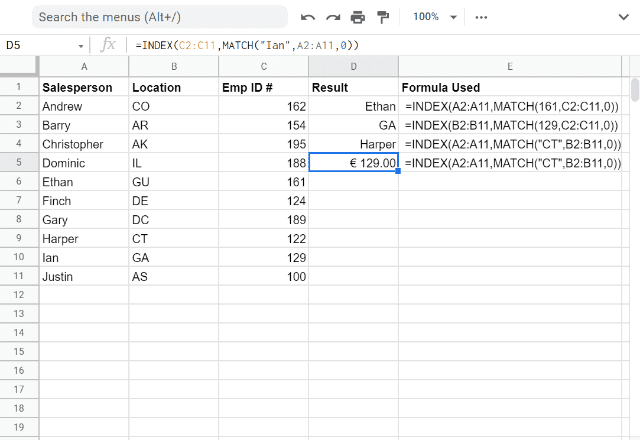In the above example, I’ve used the MATCH function to tell me which row contains Emp ID #161 within column C. The function returns a number that tells the INDEX function which row in column A to look for the Salesperson. The result is that the two functions combine to tell me the correct name (Ethan) for Emp ID #161. The function I used to do this is =INDEX(A2:A11,MATCH(161,C2:C11,0)).

As you can see, unlike VLOOKUP, the combination works even if the lookup column is not the leftmost (see the first three examples in the above screenshot). Not surprisingly, it also works like VLOOKUP, when the lookup column is the leftmost.

Let’s also see whether the functions hold up when you introduce a new column in between.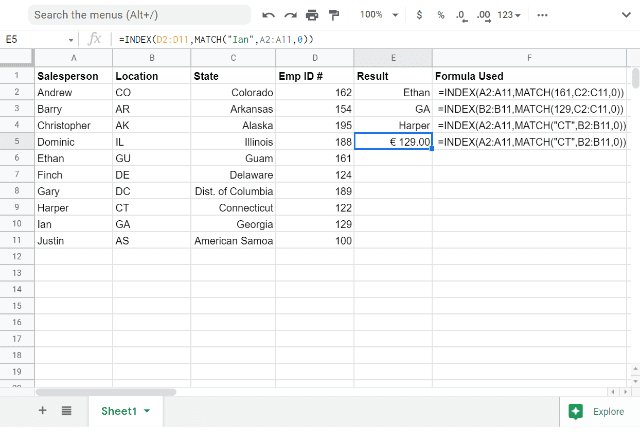Thankfully, they still work. As soon as I introduced a new column (State), Google Sheets updated the references automatically to accommodate this change.

As you’ve seen above, the MATCH and INDEX function combination is much more flexible and versatile than the already popular and powerful VLOOKUP function. But the combination of these functions can be even more powerful when you use two MATCH functions instead of just one.

### How to use INDEX function with 2 MATCH functions

You’ve now seen how useful the INDEX and MATCH combination can be. But what if you have a bi-dimensional array from which you want to get a value? In this case, you can use the MATCH function twice within the INDEX function, as shown in the example below.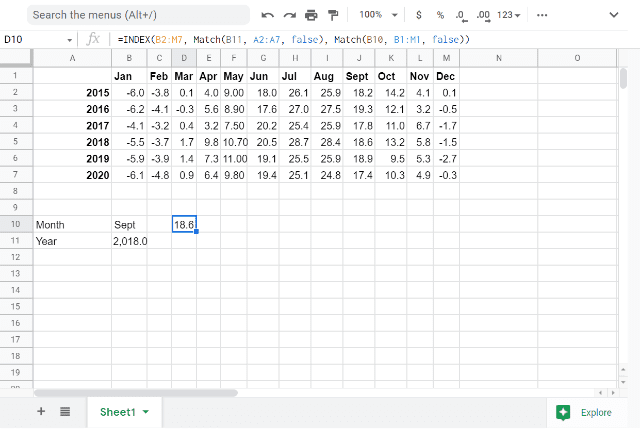In this example, you can see data on the average temperature in each month, for the years 2015-2020. If you want to pull the average temperature of a specific month of a specific year out of this array, you can use the INDEX and MATCH combination to accomplish this. The function used here is:
=INDEX(B2:M7, Match(B11, A2:A7, false), Match(B10, B1:M1, false))

As you can see, I am using the MATCH function to give me the location of the row for the year I am looking for (in this case it’s 2018, which is in the 4th row of data) as well as the column for the month I am looking for (September, in the 9th column of data). The INDEX function then takes these co-ordinates and returns the average temperature for September 2018.

By using the INDEX and MATCH functions, you can not only get a search function that is more versatile than VLOOKUP, but you can also search bi-dimensional arrays. To learn more about the INDEX function and its use, check out the following blog post: How to use the INDEX function in Google Sheets.

Editor’s note: This is a revised version of a previous post that has been updated for accuracy and comprehensiveness.